Courses

# Test: Applications Of Trigonometric Identities

## 15 Questions MCQ Test Mathematics (Maths) Class 10 | Test: Applications Of Trigonometric Identities

Description
This mock test of Test: Applications Of Trigonometric Identities for Class 10 helps you for every Class 10 entrance exam. This contains 15 Multiple Choice Questions for Class 10 Test: Applications Of Trigonometric Identities (mcq) to study with solutions a complete question bank. The solved questions answers in this Test: Applications Of Trigonometric Identities quiz give you a good mix of easy questions and tough questions. Class 10 students definitely take this Test: Applications Of Trigonometric Identities exercise for a better result in the exam. You can find other Test: Applications Of Trigonometric Identities extra questions, long questions & short questions for Class 10 on EduRev as well by searching above.
QUESTION: 1

### If 7sin2x + 3cos2x = 4 then, secx + cosecx =

Solution:

7sin2x+3cosx=4
7sin2x+3(1-sin2x)=4
7sin2x+3-3sin2x=4
4sin2x=4-3
4sin2x=1
sin2x=¼
sinx=½
Cosec x=1/sinx=2
Cos x=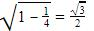Sec x= 1/cos x=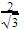Cosec x + sec x=2+QUESTION: 2

Solution:
QUESTION: 3

### If tan θ = 12/5, then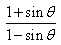is equal to

Solution:

tanθ = 12/5

so sinθ = 12/13

so

(1 + 12/13)/(1-12/13)

= 25/1 = 25

QUESTION: 4

When 0°< θ < 90°, and 2 cos θ = 1 the value of θ is​

Solution:

2cosθ = 1
cosθ = 1/2 ⇒ θ = 60 degrees

QUESTION: 5Solution:

Rationalise 1 +sinA

QUESTION: 6

The square root of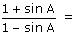​

Solution:
QUESTION: 7

If tanθ < 0, sinθ < 0, then the terminal arm of the angle lies in the quadrant

Solution:

The correct option is Option A.

If the angle is between 0 to 90 degrees then it will lies in the first quadrant.

The tanθ and sinθ have positive values, hence lies in first quadrant.

QUESTION: 8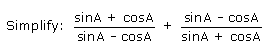Solution:
QUESTION: 9

The value of cos θ cos(90° - θ) – sin θ sin (90° - θ) is:

Solution:
QUESTION: 10

tan2A – tan2B can also be written as.

Solution:
QUESTION: 11

The value of θ for which 2 cos2θ + sinθ - 2 =; 0° < θ < 90° is:​

Solution:
QUESTION: 12

If 2 cos(A + B) = 1, and 2 sin(A –B) = 1 then the values of A and B are

Solution:

2 cos(A + B) = 1
cos(A + B) = ½
cos(A + B)=cos 60
A+B=60   …(1)
2 sin(A – B) = 1
sin(A - B)=½
sin(A - B)=sin 30
A-B = 30    …(2)
Adding 1 and 2
2A = 90
A = 45
B = 15

QUESTION: 13

If a cosθ + b sinθ = 4 and a sinθ – b cosθ = 3, then a2 + b2 is

Solution:
QUESTION: 14

If θ is an acute angle and tan θ + cot θ​ = 2, then the value of tan7θ + cot7θ is is

Solution: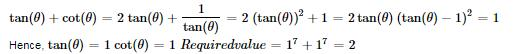QUESTION: 15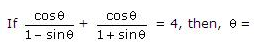Solution: